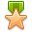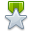×

Help with power factor calculations, how to find KW from KVA and KVARJump to latest post
1.NETA Level III
Join Date
Aug 2014
Location
Capital City
Posts
74
ReputationHelp with power factor calculations, how to find KW from KVA and KVAR

A single line feeds 2 separate loads. The first load is 1200kw at .80 pf. The second load is 800kva and 300 kvar. What is the total load on the single line feeder?

I have been racking my brain trying to figure out this question that someone else said was on the Level 4 exam. My answer is 1702kW, can anyone let me know if I am right? Here is how I figured it out:

Load 1: Easy, just correct kW for power factor. 1200 * 0.8 = 960kW

Load 2: This is where it got tricky but this is how I was able to calculate kW from kVA and kVAR, I think:

(800kVA)2 = 640000
(300kVAR)2 = 90000
640000 - 90000 = 550000
Square root (550000) = 742kW

Just looking to know if this is correct. Thanks in advance.Reply With Quote

2.Junior Member
Join Date
Jan 2015
Posts
24
Reputationkva kvar kw calculation

The equation is: KW = KVA x pf, therefore KVA = KW/pf
1200 kW = KVA x 0.8 ..... KVA = 1200/0.8 = 1500 KVA,
In other words, the 1200 kW, with pf 0.8, needs to have available 1500 KVA.

For the second question we have:
(KVA)2 = (KVAR)2 + (KW)2 .... (800)2 = (300)2 + (KW)2 (This is you got it right)

Observe that you can use the above equation to calculate the KVAR for the first question:
(1500)2 = (KVAR)2 + (1200)2
KVAR = Square root ( 2250000 - 1440000) .... KVAR = Square root(810000) = 900
Load: 1500 + 800 = 2300 KVA
1200 + 742 = 1942 KW
900 + 300 = 1200 KVARReply With Quote

3.NETA Level III
Join Date
Aug 2014
Location
Capital City
Posts
74
Reputationhow to find kvar

doh! thats what i get for not double checking my work. thanks for clearing this up for me!Originally Posted by joaogemalThe equation is: KW = KVA x pf, therefore KVA = KW/pfReply With Quote

4. kva to kw

No calculation is necessary for load 1, you are given the kW.Originally Posted by veracon0700Load 1: Easy, just correct kW for power factor. 1200 * 0.8 = 960kWReply With Quote

5.Junior Member
Join Date
Jan 2016
Posts
5
Reputationkvar kw

i found this power factor triangle to be helpful in understanding these kind of calculationsReply With Quote

6.Junior Member
Join Date
May 2017
Posts
7
ReputationOriginally Posted by veracon0700A single line feeds 2 separate loads. The first load is 1200kw at .80 pf. The second load is 800kva and 300 kvar. What is the total load on the single line feeder?

I have been racking my brain trying to figure out this question that someone else said was on the Level 4 exam. My answer is 1702kW, can anyone let me know if I am right? Here is how I figured it out:

Load 1: Easy, just correct kW for power factor. 1200 * 0.8 = 960kW

Load 2: This is where it got tricky but this is how I was able to calculate kW from kVA and kVAR, I think:

(800kVA)2 = 640000
(300kVAR)2 = 90000
640000 - 90000 = 550000
Square root (550000) = 742kW

Just looking to know if this is correct. Thanks in advance.
I guess you want to come up with all three values for both feeders to get all parameters of the load. In the case of the first load 1200KW at .8 - 1200/.8 = 1500KVA. The angle is 36.869 degrees so you have (sine) 900KVAR. Load 1 is fully described as 1500KVA, 1200KW, and 900KVAR.

Load 2 at 800KVA and 300KVAR. The angle is ARC SIN 300/800 = 22.02 degrees. Watts then is 741.619 KW. Just add them all up for the total of each parameter. The assumption is that the vars and watt flow is in the same direction. Alternatively you can use the Pythagorean theorem for load 2 - that is 800(squared) = 300(squared) + x(squared) and you get the same 741.619 (as a cross check). www.cttestset.comReply With Quote

7.Member
Join Date
May 2018
Posts
25
ReputationOriginally Posted by veracon0700A single line feeds 2 separate loads. The first load is 1200kw at .80 pf. The second load is 800kva and 300 kvar. What is the total load on the single line feeder?

I have been racking my brain trying to figure out this question that someone else said was on the Level 4 exam. My answer is 1702kW, can anyone let me know if I am right? Here is how I figured it out:

Load 1: Easy, just correct kW for power factor. 1200 * 0.8 = 960kW

Load 2: This is where it got tricky but this is how I was able to calculate kW from kVA and kVAR, I think:

(800kVA)2 = 640000
(300kVAR)2 = 90000
640000 - 90000 = 550000
Square root (550000) = 742kW

Just looking to know if this is correct. Thanks in advance.
This question threw me for a loop at first, too. I saw the first numbers so I went ahead and calculated the kVA for 1200kW at 0.80pf. I used pythagorean theorem for the 2nd part of the question and then realized that I didn't need the kVA from the first part of the question. They had already given me the real power value. Oops.Reply With Quote

8.Junior Member
Join Date
Apr 2018
Posts
16
ReputationGreat thread. I'm quite a ways off from even level 3, but like skipping ahead.Reply With Quote

electrical calculations, electrical theory, neta exam questions, neta exam tips, neta level 3, neta level 4Related Content